# Posts tagged as “combination”

## Problem

Given an integer array, your task is to find all the different possible increasing subsequences of the given array, and the length of an increasing subsequence should be at least 2 .

Example:

Input: [4, 6, 7, 7]
Output: [[4, 6], [4, 7], [4, 6, 7], [4, 6, 7, 7], [6, 7], [6, 7, 7], [7,7], [4,7,7]]


Note:

1. The length of the given array will not exceed 15.
2. The range of integer in the given array is [-100,100].
3. The given array may contain duplicates, and two equal integers should also be considered as a special case of increasing sequence.

# Solution: DFS

Time complexity: O(2^n)

Space complexity: O(n)

C++

# Problem:

A binary watch has 4 LEDs on the top which represent the hours (0-11), and the 6 LEDs on the bottom represent the minutes (0-59).

Each LED represents a zero or one, with the least significant bit on the right.

#For example, the above binary watch reads “3:25”.

Given a non-negative integer n which represents the number of LEDs that are currently on, return all possible times the watch could represent.

Example:

Input: n = 1
Return: ["1:00", "2:00", "4:00", "8:00", "0:01", "0:02", "0:04", "0:08", "0:16", "0:32"]

Note:

• The order of output does not matter.
• The hour must not contain a leading zero, for example “01:00” is not valid, it should be “1:00”.
• The minute must be consist of two digits and may contain a leading zero, for example “10:2” is not valid, it should be “10:02”.

# Solution 1:

Time complexity: O(11*59*n)

C++

Find out how many ways to assign symbols to make sum of integers equal to target S.

Example 1:

Note:

1. The length of the given array is positive and will not exceed 20.
2. The sum of elements in the given array will not exceed 1000.
3. Your output answer is guaranteed to be fitted in a 32-bit integer.

# Idea: DP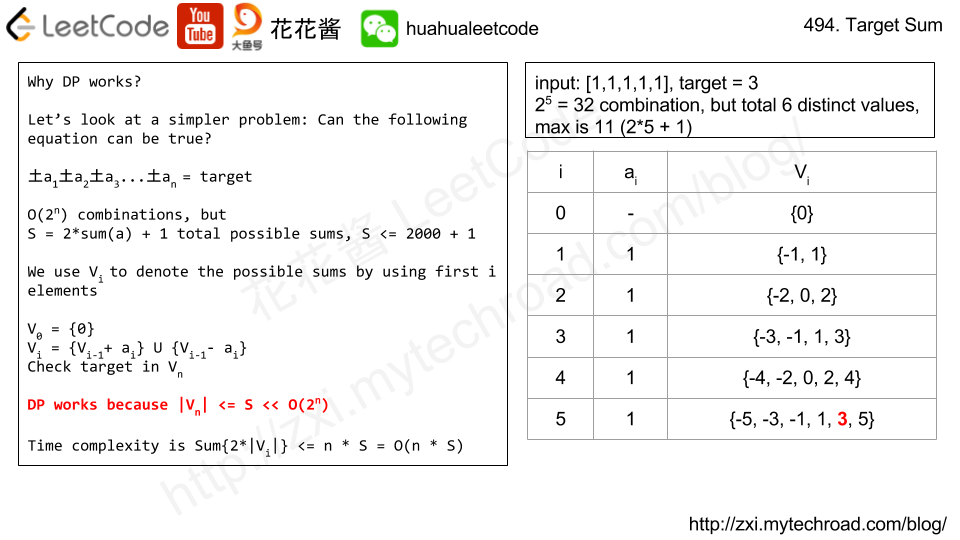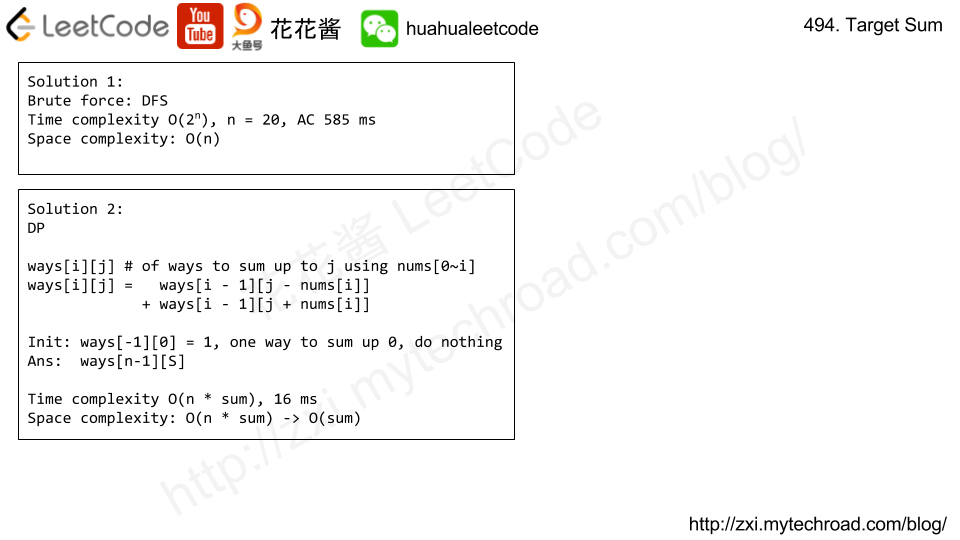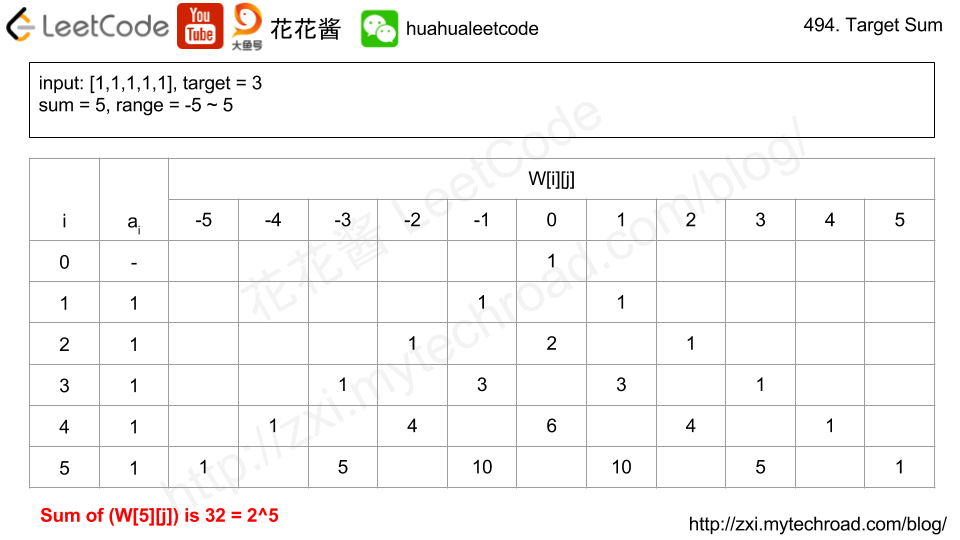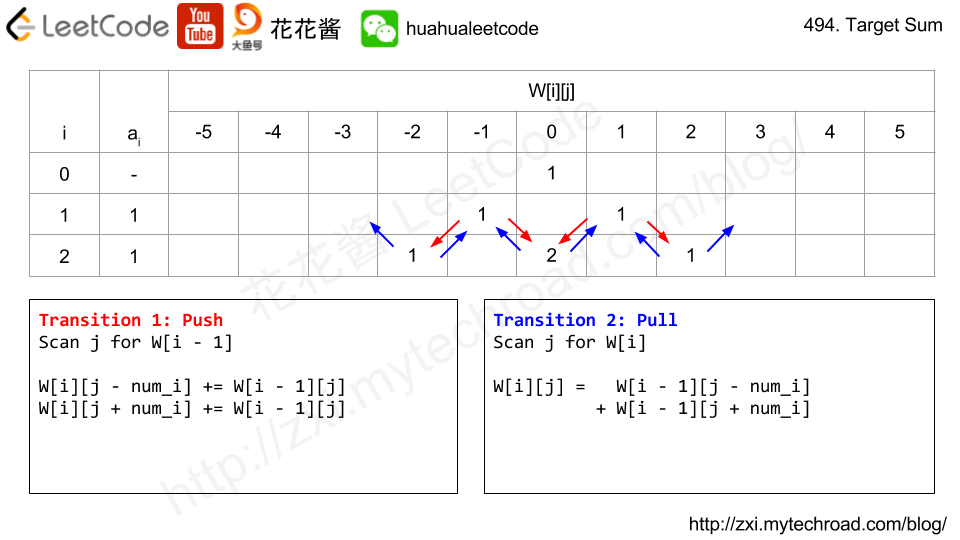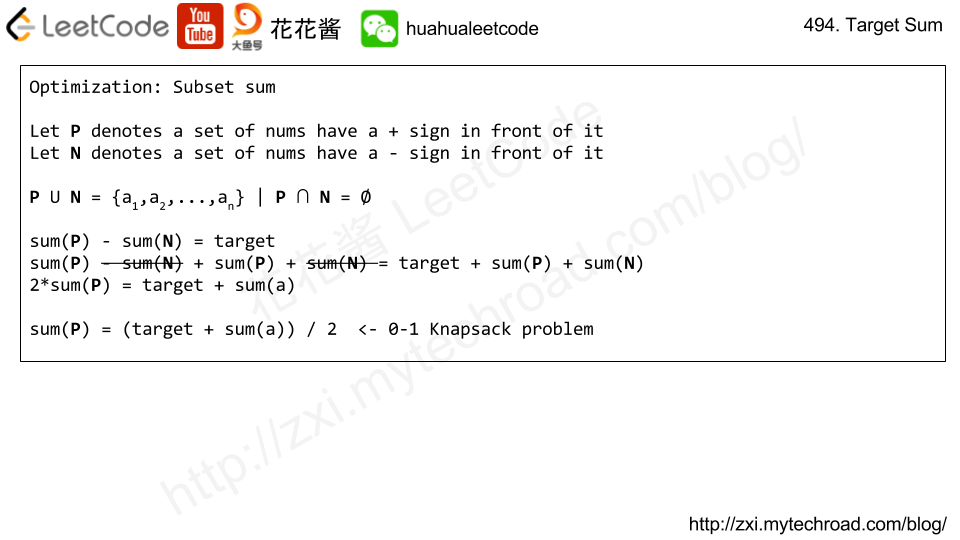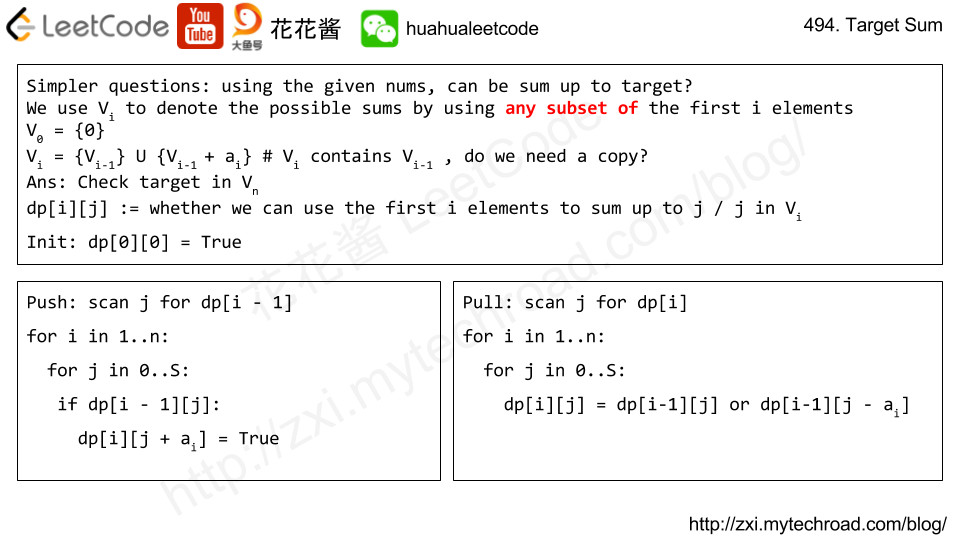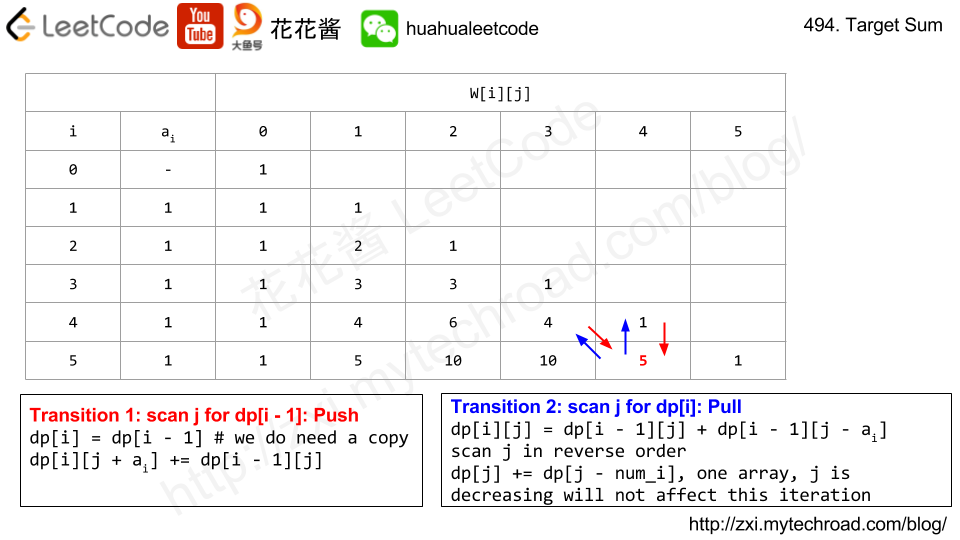# Solution 1: DP

Time complexity: O(n*sum)

Space complexity: O(n*sum)

# Solution 2: DFS

Time complexity: O(2^n)

Space complexity: O(n)

# Solution 3: Subset sum

Time complexity: O(n*sum)

Space complexity: O(sum)

## C++ w/o copy

Problem:

Given a digit string, return all possible letter combinations that the number could represent.

A mapping of digit to letters (just like on the telephone buttons) is given below.Note:
Although the above answer is in lexicographical order, your answer could be in any order you want.

Solution 1: DFS

Solution 2:  BFS

## Python

Problem:

Find all possible combinations of k numbers that add up to a number n, given that only numbers from 1 to 9 can be used and each combination should be a unique set of numbers.

Example 1:

Input: k = 3, n = 7

Output:

Example 2:

Input: k = 3, n = 9

Output:

Idea:

DFS + backtracking

bit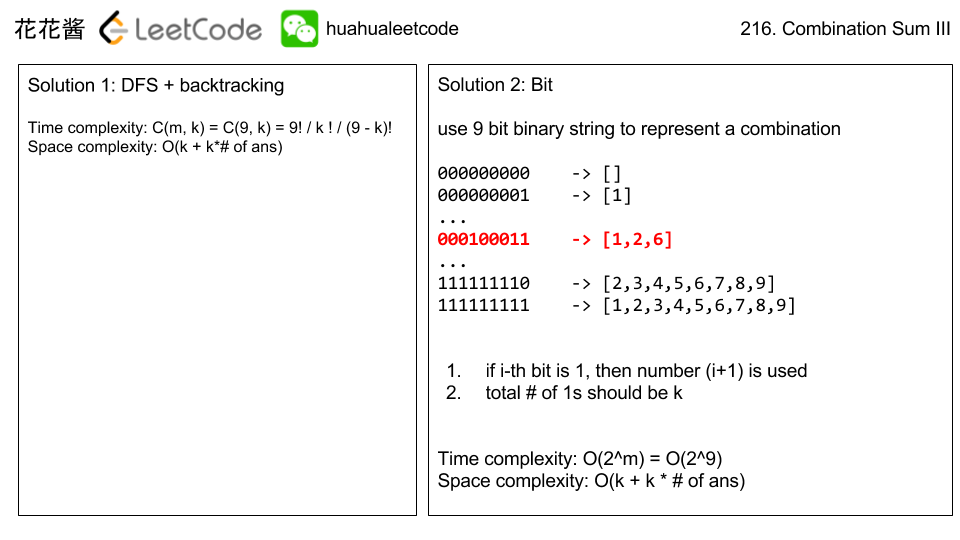Solution:

C++

C++ / binary

Python

Related problems:

Mission News Theme by Compete Themes.